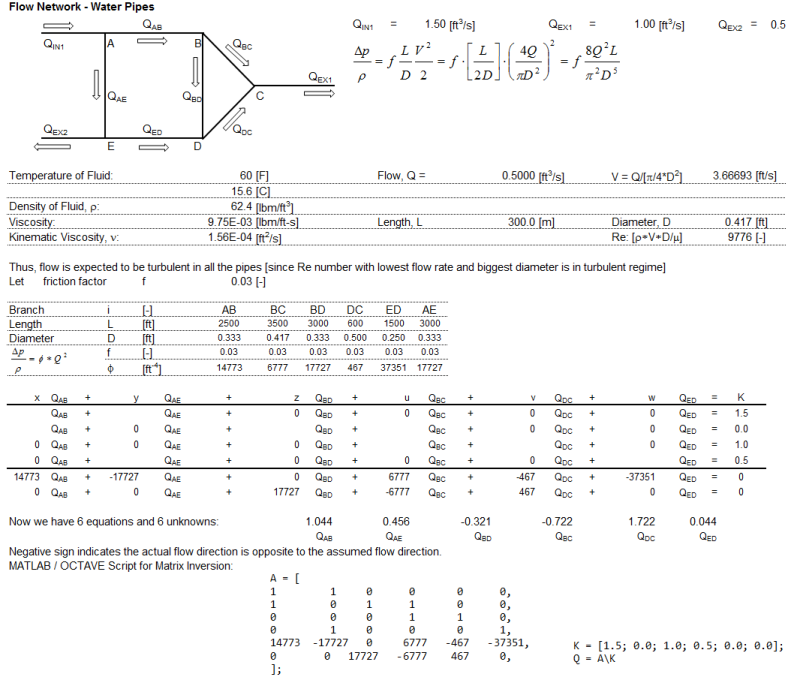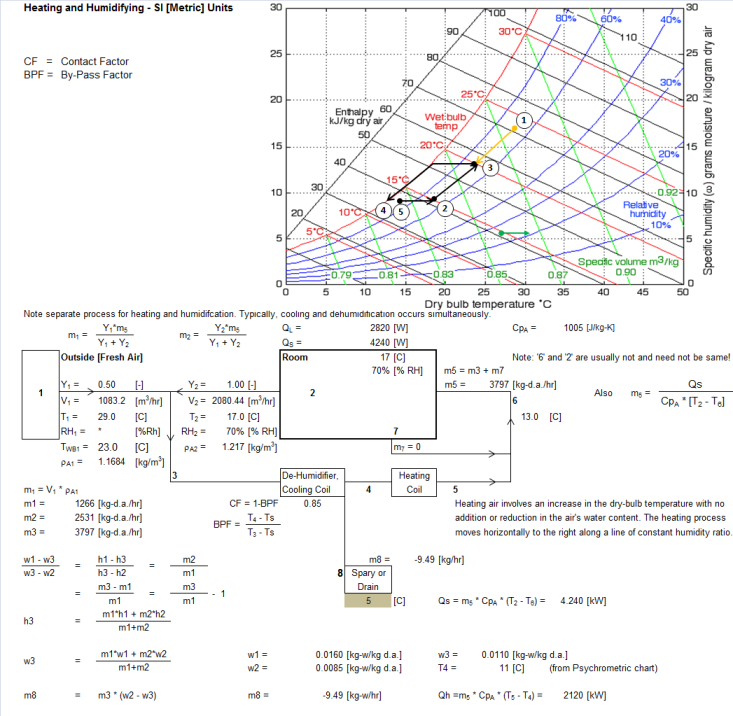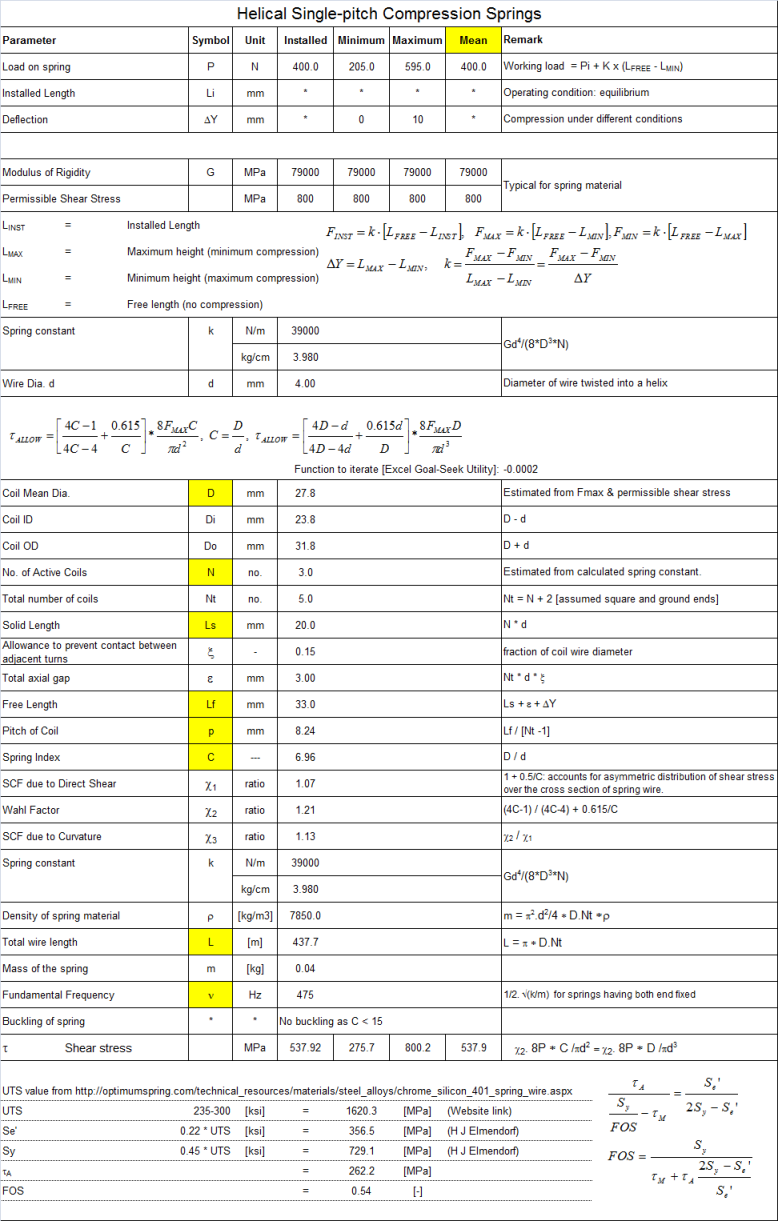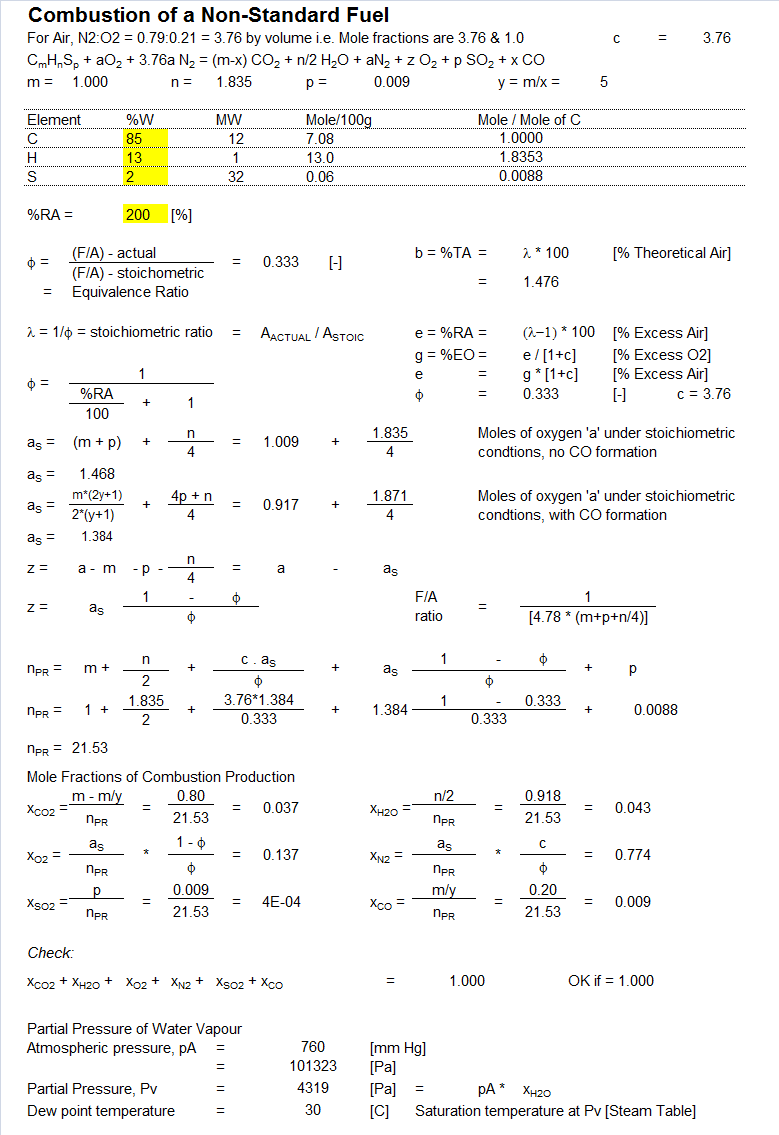• CFD, Fluid Flow, FEA, Heat/Mass Transfer

Design Calculations

### 0-D and 1-D Calculations

##### Design Calculations in Mechanical Engineering
Design calculations related to automation of repeated task can be partially automated using spreadsheet applications such as Excel and matrix inversion tools [Excel or Octave]. These design calculations also help understand the method and acceptance criteria for preliminary design - before moving on detailed calculations.
##### Psychrometry

Saturation pressure as a function of temperature: Ref: ASHRAE, input T in [K], output pSAT in [kPa]

c1 = -5800.22, c2 = -5.51626, c3 = -0.0486402, c4 = 4.17648E-5, c5 = -1.44521E-8, c6 = 6.54597

pSAT = e[c1 / T + c2 + c3 * T + c4 * T2 + c5 * T3 + c6 * Log(T)]

The dew point temperature based on TDEW = [Log(Pv / 610.78) * 241.88] / [17.558 - Log(Pv / 610.78)] where Pv in [Pa] is the vapour pressure of water in air.

##### Mixing of Moist Air Streams
 Field variables: Unit Stream-1 Stream-2 Volume flow rate - moist air: [m3/hr] Relative Humidity: [%] Dry Bulb Temperature: [°C] Saturation pressure: [Pa] Vapour pressure: [Pa] Humidity ratio: [g-vap/g-d.a.] Specific enthalpy: [J/kg-d.a.] Mass flow rate - dry air: [kg-d.a./s] Mass flow rate - vapour: [kg-vap/s] Air (moist) properties after mixing Mass flow rate (moist air): [kg/s] Specific enthalpy: [J/kg-m.a.] Humidity ratio: [J/kg-d.a.] Dry-bulb temperature: [°C] Relative humidity: [%]

#### Pipe NetworkThe flow through the network of pipes and junction can be calculated using mass balance and pressure equality. Hardy-Cross method is one of the widely used method - both for manual as well as automated process.
Go to top

## HAVC - Basic

A lumped approach to estimate the required air flow rates for maintaining an enclosure under desired condition.Go to top

#### Compression Springs

The input can vary from one design conditions to other, but the overall calculation steps remain same. In this particular case, the cells highlighted in yellow were the calculated variables.Go to top

#### Combustion - Arbitrary CompositionGo to top

#### Hydraulic DrivesThe content on CFDyna.com is being constantly refined and improvised with on-the-job experience, testing, and training. Examples might be simplified to improve insight into the physics and basic understanding. Linked pages, articles, references, and examples are constantly reviewed to reduce errors, but we cannot warrant full correctness of all content.